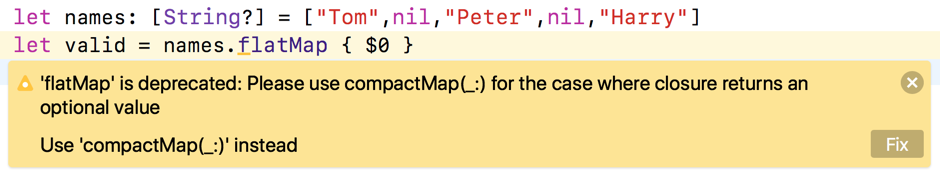## Replacing flatMap With compactMap

Swift 4.1 shipped with Xcode 9.3 and brought more changes to the Swift language and the Swift standard library. Apple intended it as a source compatible upgrade to Swift 4.0 but I was hit with one source code change that I am guessing will be widespread.

Using `flatMap` on a sequence (like an `Array`) filtering anything that maps to `nil` is now deprecated and replaced by `compactMap`.

### The Short Version Of What Is Changing

Are you using `flatMap` to remove `nil` items from an array of optionals:

``````let names: [String?] = ["Tom", nil, "Peter", nil, "Harry"]
let valid = names.flatMap { \$0 }
// ["Tom", "Peter", "Harry"]
``````

Xcode 9.3 shows a deprecation warning for using `flatMap` this way:The suggested fix from Xcode renames `flatMap` to `compactMap`:

``````let names: [String?] = ["Tom", nil, "Peter", nil, "Harry"]
let valid = names.compactMap { \$0 }
// ["Tom", "Peter", "Harry"]
``````

This applies anytime you use `flatMap` on a sequence with a closure that returns an optional. So this is also deprecated:

``````let words = ["53", "nine", "hello","0"]
let values = words.flatMap { Int(\$0) }
``````

Replacing `flatMap` with `compactMap` removes the deprecation warning:

``````let values = words.compactMap { Int(\$0) } // Returns [Int]
// [53, 0]
``````

### Tell Me More

First of all, Swift 4.1 does not deprecate all uses of `flatMap` - only one case is changing. Swift 4.0 has three situations where you can use `flatMap`:

1. Using `flatMap` on a sequence with a closure that returns a sequence:

``````Sequence.flatMap<S>(_ transform: (Element) -> S)
-> [S.Element] where S : Sequence
``````

I think this was probably the first use of `flatMap` I came across in Swift. Use it to apply a closure to each element of a sequence and flatten the result:

``````let scores = [[5,2,7], [4,8], [9,1,3]]
let allScores = scores.flatMap { \$0 }
// [5, 2, 7, 4, 8, 9, 1, 3]

let passMarks = scores.flatMap { \$0.filter { \$0 > 5} }
// [7, 8, 9]
``````

Swift 4.1 does not change this use of `flatMap`.

2. Using `flatMap` on an optional

The closure takes the non-nil value of the optional and returns an optional. If the original optional is `nil` then `flatMap` returns `nil`:

``````Optional.flatMap<U>(_ transform: (Wrapped) -> U?) -> U?

let input: Int? = Int("8")
let passMark: Int? = input.flatMap { \$0 > 5 ? \$0 : nil}
// Optional(8)
``````

Swift 4.1 does not change this use of `flatMap`.

3. Using `flatMap` on a sequence with a closure that returns an optional.

``````Sequence.flatMap<U>(_ transform: (Element) -> U?) -> U?
``````

This is the use of `flatMap` that Swift 4.1 (Xcode 9.3) replaces with `compactMap`.

``````let names: [String?] = ["Tom", nil, "Peter", nil, "Harry"]
let counts = names.compactMap { \$0?.count }
// [3, 5, 5]
``````

### What’s The Point?

In summary it seems to come down to discouraging the misuse of `flatMap` when a plain `map` will do the job:

``````let myNames: [String] = ["John", "Joe", "Jack"]

// No need to flatMap (or compactMap)
let myCounts = myNames.flatMap { \$0.count }
// [4, 3, 4]

// map is enough
let myCounts = myNames.map { \$0.count }
// [4, 3, 4]
``````

The idea of the name change to `compactMap` is to better describe what the function does. Mapping over a sequence and then compacting by removing the `nil` elements from the result. Future versions of Swift might also gives us a `compact` function for the common situation of removing `nil` values from a sequence